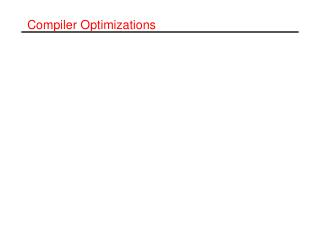DownloadDownload PresentationCompiler Optimizations

# Compiler Optimizations

Download Presentation## Compiler Optimizations

- - - - - - - - - - - - - - - - - - - - - - - - - - - E N D - - - - - - - - - - - - - - - - - - - - - - - - - - -
##### Presentation Transcript

1. Compiler Optimizations

2. Loop interchange Do j=1,n do i=1,m b(i,j)=5.0 enddo enddo Do i=1,n do j=1,m b(i,j)=5.0 enddo enddo

3. Reuse • Programs exhibit reuse • Within loop • Across loops DO I = 2, N-1 B[I] = (A[I-1]+A[I+1]) / 2 END DO DO I = 2, N-1 A[I] = B[I] END DO Reuse within loop Reuse across loops

4. Locality • Reuse can lead to cache hits if • Cache capacity is large enough (reuse distance) • No cache conflicts occur.

5. Reuse distance • Definition (reference and memory access) • A reference is a read or a write in the source code, while a memory access is one particular execution of that read or write. • Definition (reuse pair and reuse distance) • A reuse pair (r1; r2) is a pair of memory accesses in a memory access stream, which touch the same memory location, without intermediate accesses to that location. The reuse distance of a reuse pair (r1; r2) is the number of unique memory locations accessed between references r1and r2.

6. Strip-mining do i=1,n do j=1,n ... = a(i,j) enddo enddo do i=1,n do jj=1,n,B do j=jj,min(jj+B-1,n) ... = a(i,j) enddo enddo enddo

7. Tiling (Strip-mining & loop interchange) do jj=1,N,B do ii=1,N,B do t=1,T do j=jj,min(jj+B-1,N) do i=ii,min(ii+B-1,N) ... = a[i,j] end do end do end do end do end do do t=1,T do j=1,N do i=1,N ... = a[i,j] end do end do end do

8. Data transformations • Alignment • Array padding • Array element reordering • Array merging

9. Alignment • Align a data structure such that it begins at a cache line boundary. • Useful for cache-line-sized data structures. • May also help to reduce false sharing.

10. Array Padding • Increases size of inner array dimension. integer a[256,256] do j=1,N do i=1,N ... = a[i,j]+ a[i,j+1] end do end do integer a[260,256] .... j i Assumption: 64 lines á 4 words

11. Array Element Reordering • Modifies storage order for elements integer A[256,512] DO I = 1, 256 DO J = 1, 512 ...=A[I,J] ... END DO END DO integer A[512,256] DO I = 1, 256 DO J = 1, 512 ...=A[J,I] ... END DO END DO

12. Array Merging • Interleaves data from multiple arrays integer B, A DO I = 2, N-1 ... B[I]=... A[I]... ... END DO integer C DO I = 2, N-1 ... C[2*I-1]=... C[2*I]... ... END DO

13. Summary • Loop transformations and data transformations can improve locality. • Elimination of conflicts and reduction of reuse distance. • Legality of loop transformations depends on dependences. • Data transformations are always legal as long as all references can be adapted. • Profitability of transformation is extremely difficult to predict.